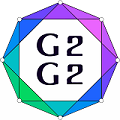#Graphs and Groups, Geometries and GAP (G2G2) Summer School - External Satellite Conference of 8ECM

27 June 2021 to 3 July 2021
Rogla, Slovenia
UTC timezone

## The nonexistence of distance-regular graphs with intersection array {56,42,20;1,6,28} in which some local subgraph is the 7x8-grid.

Not scheduled
20m
Rogla, Slovenia

#### Rogla, Slovenia

Hotel Planja, Rogla 1, 3214 Zreče, Slovenia
Oral presentation

### Speaker

Mikhail Golubyatnikov (Institute of Mathematics and Mechanics, Ural Branch of the Russian Academy of Sciences)

### Description

There is an infinite sequence of formally self-dual classical distance-regular graphs $\Gamma$ with classical parameters $b=2$, $\alpha=1$, $\beta=n-1$, $v=n^3$ $(n>5)$.

If $n$ is a power of $2$, then there exists a distance-regular graph $\Gamma$ with intersection array $\{7(n-1),6(n-2),4(n-4);1,6,28\}$ and each distance-regular graph with these parameters is a bilinear forms graph. Moreover, all these graphs are locally grid.

We consider graphs $\Gamma$ with intersection array $\{7(n-1),6(n-2),4(n-4);1,6,28\}$, where $n$ is not a power of 2. By the results of Metsch, if $n\ge 71$, then graphs with intersection array $\{7(n-1),6(n-2),4(n-4);1,6,28\}$ don't exist.

If $n=6$, then the intersection array of $\Gamma$ is $\{35,24,8;1,6,28\}$. The nonexistence of a graph with this intersection array was proved by A. Jurishich and J. Vidali. The case when $n=7$ was ruled out by I.N. Belousov and A.A. Makhnev. The proof was based on counting of some triple intersection numbers.

In this work, we consider the case $n=9$. In this case, there are $6$ admissible spectra for the integral local subgraph. One of them is relate to the $7\times 8$-grid.

We prove that a distance-regular graph with intersection array $\{56,42,20;1,6,28\}$ does not exist, if some its local subgraph is the $7\times 8$-grid.

Acknowledgments. The work is supported by Russian Science Foundation (project 19-71-10067).

### Primary author

Mikhail Golubyatnikov (Institute of Mathematics and Mechanics, Ural Branch of the Russian Academy of Sciences)